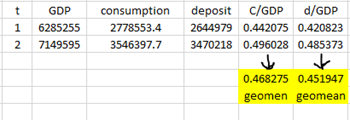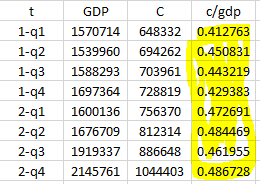# Calibration from data: stock variables and quarterly data

Hi everyone. I have a question about model calibration. I am a newbie and I apologize for asking this question. I have read Professor jpfeifer’s paper (A Guide to Specifying Observation Equations for the
Estimation of DSGE Models) But I don’t know exactly whether I am doing it right or not. Please clear me from this doubt.

I want to take help from the data and extract the parameters from the steady state of the model (Reverse the usual method)
. I divide all endogenous variables (except the rates) in the steady state by the output: (C_ss/Y_ss , I_ss/Y_ss , K_ss/ Y_ss , …)

I am dealing with two types of variables: stock variables (such as capital, deposit, loan …) and flow variables (such as output, consumption, …)

If I use annual data I can figure out how to calibrate. For example, if I have only two periods in the model And the data is as follows:If the variables are flow type and the data is quarterly, there is no problem. For example, by having quarterly GDP and consumption data, we can compute the to consumption to production ratio: The geometric mean of the yellow column is equal to : C_ss/Y_ss= 0.4960The problem arises when one of the variables is stock (for example deposit (d)) and one of the variables is flow (GDP) Also, the data is quarterly:Do I have to divide the deposit variable of each quarter by the GDP variable in the same quarter?:
C2/B2 = 1.453051
C3/B3 =1.575709
C4/B4 =1.565667

And take the geometric mean from the results obtained in the D column (=1.64)
Accordingly, I calibrate based on whether my data is quarterly or annual like this:
Quarterly: d/GDP= 1.64
Annualy: d/GDP = 0.45
is it correct?

Yes, in an annual model a stock to GDP ratio is smaller by a factor of 4, because you divide by the annual flow of GDP, which is four times bigger.

Hello dear Professor,

i need help please. I heard about a simulation that exists capable of knowing the dynamics before and without shock. For example, we want to assess the impact of a pandemic on economic growth. This simulation can manage to know how growth would have behaved without a pandemic and with a pandemic. In short, I would like to know how to go about it at this level, the code written in Matlab which makes it possible to achieve this result. I need your help please

Pretty much any structural model should allow you to do such counterfactuals.